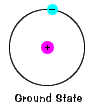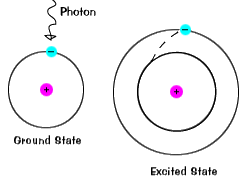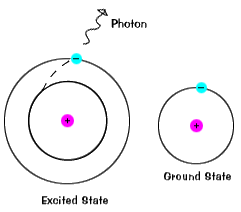# National Aeronautics and Space Administration

## Goddard Space Flight Center## Atoms and Light Energy

 The study of atoms and their characteristics overlap several different sciences. Chemists, Physicists, and Astronomers all must understand the microscopic scale at which much of the Universe functions in order to see the "bigger picture".

### Inside the Atom

Just like bricks are the building blocks of a home, atoms are the building blocks of matter. Matter is anything that has mass and takes up space (volume). All matter is made up of atoms. The atom has a nucleus, which contains particles of positive charge (protons) and particles of neutral charge (neutrons). Surrounding the nucleus of an atom are shells of electrons - small negatively charged particles. These shells are actually different energy levels and within the energy levels, the electrons orbit the nucleus of the atom.The ground state of an electron, the energy level it normally occupies, is the state of lowest energy for that electron.
 There is also a maximum energy that each electron can have and still be part of its atom. Beyond that energy, the electron is no longer bound to the nucleus of the atom and it is considered to be ionized.When an electron temporarily occupies an energy state greater than its ground state, it is in an excited state. An electron can become excited if it is given extra energy, such as if it absorbs a photon, or packet of light, or collides with a nearby atom or particle.

### Light Energy

Each orbital has a specific energy associated with it. For an electron to be boosted to an orbital with a higher energy, it must overcome the difference in energy between the orbital it is in, and the orbital to which it is going. This means that it must absorb a photon that contains precisely that amount of energy, or take exactly that amount of energy from another particle in a collision.

The illustrations on this page are simplified versions of real atoms, of course. Real atoms, even a relatively simple ones like hydrogen, have many different orbitals, and so there are many possible energies with different initial and final states. When an atom is in an excited state, the electron can drop all the way to the ground state in one go, or stop on the way in an intermediate level.Electrons do not stay in excited states for very long - they soon return to their ground states, emitting a photon with the same energy as the one that was absorbed.

### Identifying Individual Types of Atoms

Transitions among the various orbitals are unique for each element because the energy levels are uniquely determined by the protons and neutrons in the nucleus. We know that different elements have different numbers of protons and neutrons in their nuclei. When the electrons of a certain atom return to lower orbitals from excited states, the photons they emit have energies that are characteristic of that kind of atom. This gives each element a unique fingerprint, making it possible to identify the elements present in a container of gas, or even a star.

We can use tools like the periodic table of elements to figure out exactly how many protons, and thus electrons, an atom has. First of all, we know that for an atom to have a neutral charge, it must have the same number of protons and electrons. If an atom loses or gains electrons, it becomes ionized, or charged. The periodic table will give us the atomic number of an element. The atomic number tells us how many protons an atom has. For example, hydrogen has an atomic number of one - which means it has one proton, and thus one electron - and actually has no neutrons.

### For the Student

Based on the previous description of the atom, draw a model of the hydrogen atom. The "standard" model of an atom is known as the Bohr model.

Different forms of the same chemical element that differ only by the number of neutrons in their nucleus are called isotopes. Most elements have more than one naturally occurring isotope. Many more isotopes have been produced in nuclear reactors and scientific laboratories. Isotopes usually aren't very stable, and they tend to undergo radioactive decay until something that is more stable is formed. You may be familiar with the element uranium - it has several unstable isotopes, U-235 being one of the most commonly known. The 235 means that this form of uranium has 235 neutrons and protons combined. If we looked up uranium's atomic number, and substracted that from 235, we could calculate the number of neutrons that isotope has.

Here's another example - carbon usually occurs in the form of C-12 (carbon-12) , that is, 6 protons and 6 neutrons, though one isotope is C-13, with 6 protons and 7 neutrons.

### For the Student

Use the periodic table and the names of the elements given below to figure out how many protons, neutrons and electrons they have. Draw a model of an atom of the following element: silicon-28, magnesium-24, sulphur-32, oxygen-16, and helium-4.

### For the Student

Using the text, define the following terms: energy levels, absorption, emission, excited state, ground state, ionization, atom, element, atomic mass, atomic number, isotope.

### A Optional Note on the Quantum Mechanical Nature of Atoms

While the Bohr atom described above is a nice way to learn about the structure of atoms, it is not the most accurate way to model them.

Although each orbital does have a precise energy, the electron is now envisioned as being smeared out in an "electron cloud" surrounding the nucleus. It is common to speak of the mean distance to the cloud as the radius of the electron's orbit. So just remember, we'll keep the words "orbit" and "orbital", though we are now using them to describe not a flat orbital plane, but a region where an electron has a probability of being.

Electrons are kept near the nucleus by the electric attraction between the nucleus and the electrons. Kept there in the same way that the nine planets stay near the Sun instead of roaming the galaxy. Unlike the solar system, where all the planets' orbits are on the same plane, electrons orbits are more three-dimensional. Each energy level on an atom has a different shape. There are mathematical equations which will tell you the probability of the electron's location within that orbit.

Let's consider the hydrogen atom, which we already drew a Bohr model of.Probable locations of the electron in the ground stateof the Hydrogen atom. What you're looking at in these pictures are graphs of the probability of the electron's location. The nucleus is at the center of each of these graphs, and where the graph is lightest is where the electron is most likely to lie. What you see here is sort of a cross section. That is, you have to imagine the picture rotated around the vertical axis. So the region inhabited by this electron looks like a disk, but it should actually be a sphere. This graph is for an electron in its lowest possible energy state, or "ground state."

 To the right is an excited state of hydrogen. Notice that at the center, where the nucleus is, the picture is dark, indicating that the electron is unlikely to be there. The two light regions, where the electron is most likely to be found, are really just one region. Remember, you have to mentally rotate this around a vertical axis, so that in three dimensions the light region is really doughnut shaped.Probable locations of the electron in an excited stateof Hydrogen.

The text and images in this section were adapted from Dave Slaven's page on The Atom (see References below).

### Reference URLs:

The Periodic Table
http://www.webelements.com/

Back to the Main Spectra Unit MenuA service of the High Energy Astrophysics Science Archive Research Center (HEASARC), Dr. Alan Smale (Director), within the Astrophysics Science Division (ASD) at NASA/GSFC